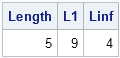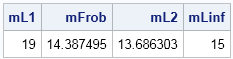Did you know that SAS/IML 12.1 provides built-in functions that compute the norm of a vector or matrix? A vector norm enables you to compute the length of a vector or the distance between two vectors in SAS. Matrix norms are used in numerical linear algebra to estimate the condition number of a matrix, which is important in understanding the accuracy of numerical computations.

Vector norms are probably familiar to many readers. The vector norms that are used most often in practice are as follows:

• The L1 or "Manhattan" norm, which is the sum of the absolute values of the elements in a vector
• The L2 or "Euclidean" norm, which is the square root of the sum of the squares of the elements
• The L or "Chebyshev" norm, which is the maximum of the absolute values of the elements

The following program demonstrates these vector norms:

```proc iml; u = {-2, 4, -2, 1}; Length = norm(u); /* Euclidean norm = sqrt(ssq(u)) */ L1 = norm(u, "L1"); /* L1 norm = sum(abs(u)) */ Linf = norm(u, "Linf"); /* infinity norm = max(u) */ print Length L1 Linf;```Matrix norms might be less familiar to some readers. A matrix norm tells you how much a matrix can stretch a vector. For a matrix A, the matrix norm of A is the maximum value of the ratio || Av || / || v ||, where v is any nonzero vector. The matrix norm of A is denoted || A || and depends on (or "subordinate to") a vector norm. The matrix norms that are used most often in practice are as follows:

• The matrix 1-norm, which is the maximum over the sum of the absolute values of each column
• The Frobenius norm, which is the square root of the sum of the squares of the elements.
• The matrix 2-norm, which is the largest singular value of the matrix. The 2-norm is also called the spectral norm.
• The matrix ∞-norm, which is the maximum over the sum of the absolute values of each row

Both the Frobenius norm and the matrix 2-norm are subordinate to the vector 2-norm. It turns out that the Frobenius norm is equal to the square root of the sum of squares of the singular values of a matrix. Thus it is similar to the matrix 2-norm: the 2-norm is the largest singular value, whereas the Frobenius norm involves all of the singular values.

Here are some simple SAS/IML computations for the matrix that appears in the Wikipedia article on matrix norm:

```A = {3 5 7, 2 6 4, 0 2 8}; mL1 = norm(A, "L1"); /* = max(A[+,]) */ mFrob = norm(A, "Frobenius"); /* = sqrt(A[##]) */ mL2 = norm(A, "L2"); /* = largest singular value */ mLinf = norm(A, "Linf"); /* = max(A[,+]) */ print mL1 mFrob mL2 mLinf;```As I said, matrix norms give a measure of how much a nonzero vector can be stretched when transformed by a matrix. A matrix with a unit norm does not increase the length of vectors. A matrix with a large norm is called an ill-conditioned matrix. An ill-conditioned matrix can take a unit-length vector and stretch it by a large amount. Thus small uncertainties in the domain vector get magnified and lead to large uncertainties in the range. Consequently, matrix norms are used in numerical linear algebra to study how errors propagate during matrix computations.

Share